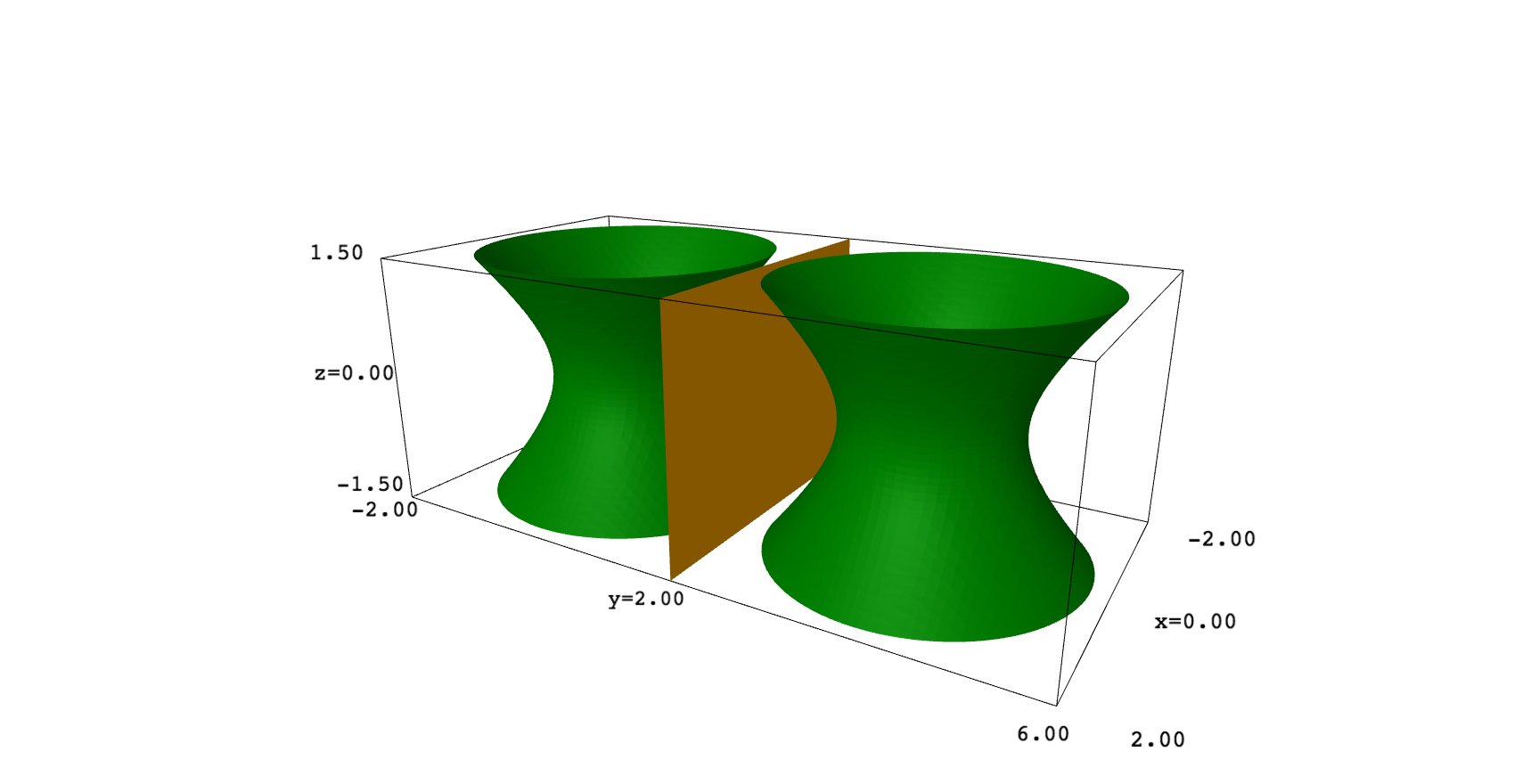# How to get the points from a contour plot object?

I've defined a plot object for the hyperboloid in R^(-1,1).

hyperboloid = region_plot(-x^2+y^2 == 1, (x,-3,3),(y,-3,3))


I want to reflect this hyperboloid across a plane, and to do that I can't think of a better way than to somehow extract the points from the ContourPlot object behind the hyperboloid and then apply a reflection transformation to each point, then plot the points I get back. How do I get the points backing the plot? If that's not possible, or if there is a better way, how can I achieve my desired reflection?

edit retag close merge delete

Sort by » oldest newest most votedI don't understand what it is $R^{(-1,1)}$ and, IMHO, an hyperboloid is a surface, so it should be traced in 3D. In such a case, you can play with translations, scales and rotations to apply reflections. For example, this code plots a one sheet hyperboloid and mirrors it on the plane $y=2$:

var("x,y,z")
p = implicit_plot3d(x^2+y^2-z^2==1, (x,-2,2), (y,-2,2), (z,-1.5,1.5), color="green")
q = p.translate([0,-2,0]).scale([1,-1,1]).translate([0,2,0])
plane = implicit_plot3d(y==2,(x,-2,2), (y,-2,2), (z,-1.5,1.5), color="orange")
show(p+q+plane)more